Home > A2C > Chapter 2 > Lesson 2.1.1 > Problem2-14

2-14.
1. Write each expression below in a simpler form. Homework Help ✎

1.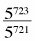2.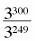3.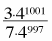4.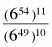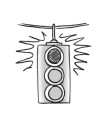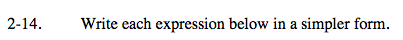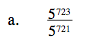Remember what exponent means.

52 or 25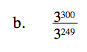See part (a).

351

$\frac{3\cdot4^{4}}{7}$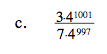Notice that the 7 and 3 are not being raised to any power.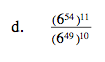How many 6's will there be if you multiply eleven 654's together: 654 · 654 · 654 ... ?

6104